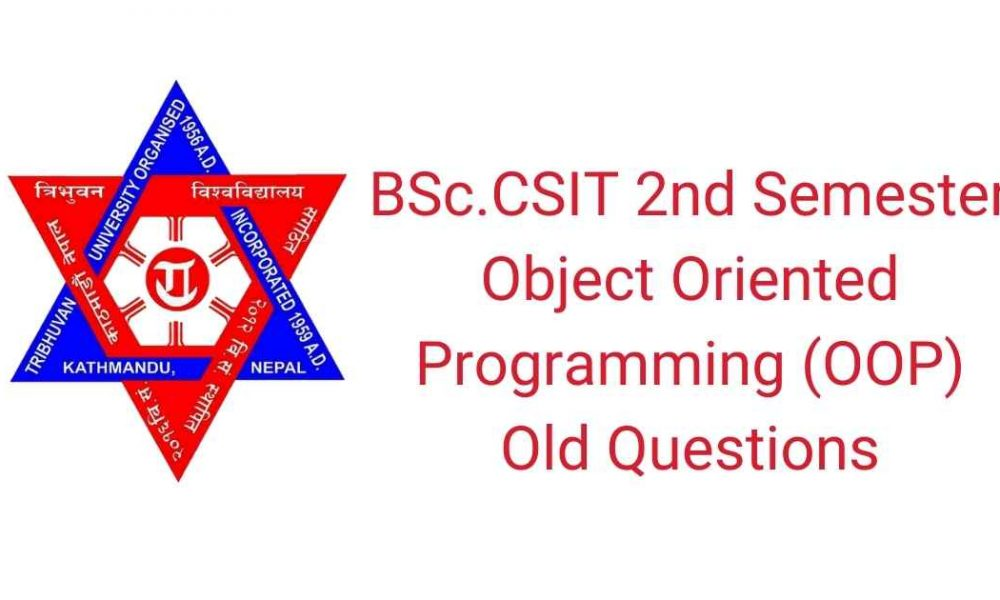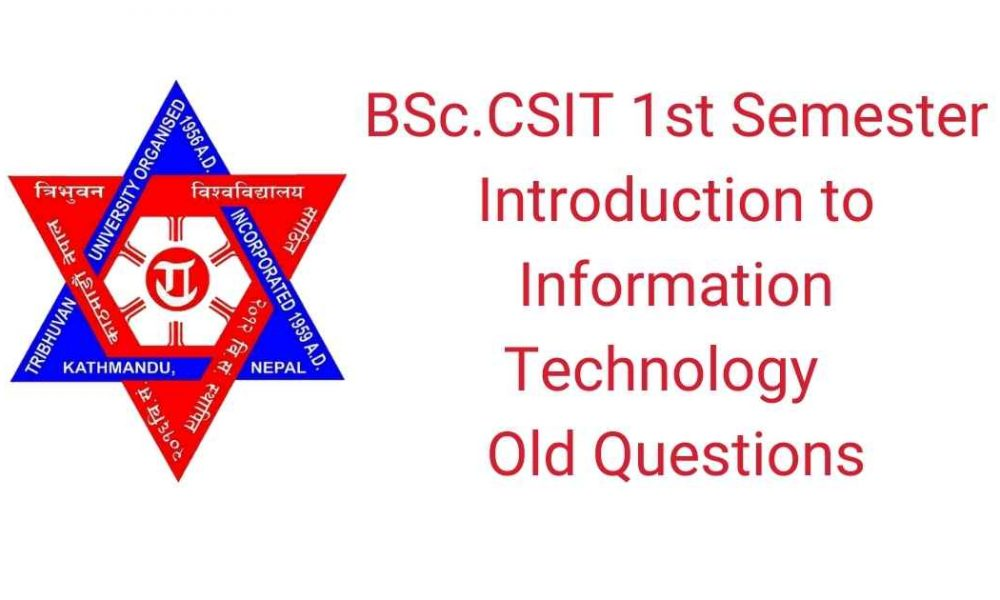# BSc.CSIT 2nd Semester Old Questions – Object-Oriented Programming## Subject: Object-Oriented Programming

### BSc.CSIT Second semester OOP Questions of Year: 2066

Attempt any two questions: (2×10=20)

1. Explain in detail the following principles of Object-Oriented Programming.
i) Data encapsulation and data hiding.
ii) Inheritance and Polymorphism.
iii) Abstraction
2. Why constructor and destructor are required on Object Oriented Programming? Explain with suitable
example.
3. Define a student class (with necessary constructors and member functions) in Object Oriented
Programming (abstract necessary attributes and their types). (Write a complete code in C++ programming
language).
 Derive a Computer Science and Mathematics class from student class adding necessary
attributes (at least three subjects).
 Use these classes in a main function and display the averages marks of computer science and
mathematics students.

Section B

Attempt any eight questions: (8×5=40)

1. What is type casting? Explain with suitable example.
2. Write a program to compute subtraction of two complex numbers using operator overloading.
3. Why exception handling is required? Explain with suitable example.
4. Differentiate between super class and sub class with suitable examples.
5. Write a program in C++ to count a number of words in a line of text.
6. Differentiate between function overriding and function overloading. Explain with suitable example.
7. Explain the role of polymorphism in Object Oriented Programming.
8. Explain the different types of class access specifiers.
9. Write a program to find the cube of given integer using inline function.
10. Write a program to convert centigrade into Fahrenheit temperature.

### Year : 2067 BSc.CSIT Second semester OOP Questions

Attempt any two questions: (2×10=20)

1. Discuss the feature of the Object-Oriented Programming. Differentiate between Object Oriented
Programming and Procedural Oriented Programming.
2. What is constructor? Explain their types? Discuss user defined parameterized constructor with suitable
example.
3. Define a clock class (with necessary constructors and member functions) in Object Oriented Programming
(abstract necessary attributes and their types). (Write a complete code in C++ programming language).
 Derive a wall_clock class from clock class adding necessary attributes.
 Create two objects of wall_clock class with all initial state to 0 or NULL.

Section B

Attempt any eight questions: (8×5=40)

1. How can you classify objects? Why dynamic objects are needed?
3. Why type conversion is necessary in OOP? Explain with example, the type conversion routine.
4. What is Inheritance? Explain their types with their suitable examples.
5. What is friend function? Why it is used in OOP? Explain with an example.
6. What is container class? Differentiate container class from inheritance.
7. Explain the role of virtual function in Object Oriented Programming.
8. Explain about “this” pointer with suitable example.
9. Write a program to find the square of given integer using inline function.
10. Write a program to convert feet into meter.

### BSc.CSIT Second semester OOP Questions of Year : 2068

Attempt any two questions: (2×10=20)

1. What are the main feature of the Object-Oriented Programming. Explain with suitable practical examples.
2. Explain the role of constructor and destructor in Object-Oriented Programming. Discuss user defined
parameterized constructor with suitable example.
3. Define a Shape class (with necessary constructors and member functions) in Object Oriented Programming
(abstract necessary attributes and their types). (Write a complete code in C++ programming language).
 Derive Triangle and Rectangle classes from Shape class adding necessary attributes.
 Use these classes in a main function and display the area of triangle and rectangle.

Section B

Attempt any eight questions: (8×5=40)

1. Why dynamic object is needed? Explain with suitable example.
3. Write a C++ program containing a possible exception. Use a try block to throw it and a catch
block to handle it properly.
4. Differentiate between base class and derived class with suitable examples.
5. Differntiate between private, public and protected variable with suitable example.
6. Differentiate container class from inheritance. Explain with suitable example.
7. Explain the role of polymorphism in Object Oriented Programming.
8. Explain about “this” pointer with suitable example.
9. Write a program to find the square root of given integer using inline function.
10. Write a program to convert inch into centimeter.

### BSc.CSIT Second semester OOP Questions of 2069

Attempt any two questions: (2×10=20)

1. Differentiate between structural programming approach and object oriented programming approach.
Explain inheritance and polymorphism with example.
2. How is a member function of a class defined? Define friend function. What are the merits and demerits of
using friend function? Explain.
3. Define constructor. List some of the special properties of the constructor functions.

Section B

Attempt any eight questions: (8×5=40)

1. Explain the do| while structure.
2. Explain the inline function with example.
3. How is dynamic initialization of objects achieved?
4. What are the importance of destructors?
5. What is an operator function? Explain with syntax.
6. Explain with example, how can you create space for array of objects using pointers?
7. Explain the features of I/O system supported by C++.
8. Differentiate between overloaded functions and function templates.
9. What are the main advantages of using exception handling mechanism in a program?

### Year : 2070 BSc.CSIT Second semester OOP Questions

Attempt any two questions
1.) Write any four features of object-oriented programming. Differentiate between operator

: (2*10=20)

2.) Why do we need the preprocessor directive # include < io stream >? Describe the major parts of
a C++ program .
3.) What do you mean by overloading of a function? When do we use this concept? Explain with
example.

Section B

Attempt any eight questions
4.) What do you mean by dynamic initialization of variables?

: (8*5=40)

5.) Explain with example of an inline function.
6.) What is virtual function? Explain.
7.) Differentiate between structure and class in terms of access modifier.
8.) What are the characteristics of constructor?
10.)Explain the function templates with example.
11.)How can we define our functions inside the namespace and use them outside?
12.)Write the syntax and use of get line () and write () functions.
13.)Explain the friend function with its syntax.

### Year: 2071 OOP Questions of BSc.CSIT Second semester

Attempt any two questions: (2×10=20)

1. Why do we need object oriented programming? How can we use inheritance to reuse already written and
tested code in programs? Discuss with suitable example. (3+3+4)
2. Discuss features of class and object. Design a class to represent a bank account with data members name,
account-number, account-type, and balance and functions to assign initial values, to deposit an amount, to
withdraw an amount after checking balance, and to display the name and balance. (4+6)

Section B

Attempt any eight questions: (8×5=40)

1. Discuss polymorphism with example.
2. What is library function? How is it different from user defined function?
3. What is looping? Differentiate while loop with do-while loop with example.
4. Discuss the use of inline function with example.
5. What is constructor? Differentiate it with destructor.
6. Explain about accessing a member function outside a class with example.
7. Write a program that decreases an integer value by 1 (one) by overloading-operator.
8. Differentiate between abstract base classes and concrete classes.
9. What is function template? Differentiate it with class template.
10. Write a program to show exception handling.

#### Pooja Karki

Pooja is Web Developer and Content Writer. She is fond of anything that is related to writing and coding and really passionate about her work. Her aim is to reach the goals and doing everything with a smile. Besides that, she loves traveling and reading novels.Previous Post

### BSc.CSIT 1st Semester Introduction to Information Technology Old QuestionsNext Post